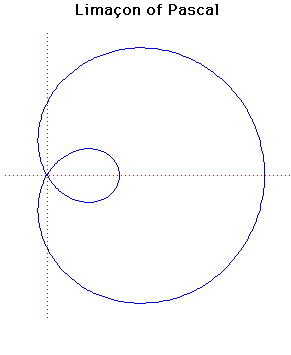# Limacon of PascalCartesian equation:
(x2 + y2 - 2ax)2 = b2(x2 + y2)
Polar equation:
r = b + 2a cos(θ)

Click below to see one of the Associated curves.

Click THIS LINK to experiment interactively with this curve and its associated curves.

Limacon of Pascal was discovered by Étienne Pascal (father of Blaise Pascal) and named by another Frenchman Gilles-Personne Roberval in 1650 when he used it as an example of his methods of drawing tangents i.e. differentiation.

The name 'limacon' comes from the Latin limax meaning 'a snail'. Étienne Pascal corresponded with Mersenne whose house was a meeting place for famous geometers including Roberval.

Dürer should really be given the credit for discovering the curve since he gave a method for drawing the limacon, although he did not call it a limacon, in Underweysung der Messungpublished in 1525.

When b = 2a then the limacon becomes a cardioid while if b = a then it becomes a trisectrix. Notice that this trisectrix is not the Trisectrix of Maclaurin.

If b ≥ 2a then the area of the limacon is (2a2 + k2)π. If b = a (the case drawn above with a = b = 1) then the area of the inner loop is a2(π - 3√3/2) and the area between the loops is a2(π + 3√3).

The limacon is an anallagmatic curve.

The limacon is also the catacaustic of a circle when the light rays come from a point a finite (non-zero) distance from the circumference. This was shown by Thomas de St Laurent in 1826.
Other Web site:

JOC/EFR/BS January 1997